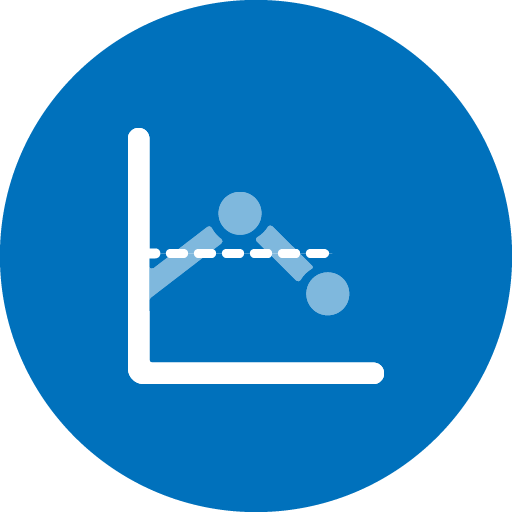#getAggregatedValueFromTS v1.1.9

by Waylay

Get an aggregated value from the time series database for the past minutes. Resource is etiher provided from the input field, raw data or from the resource associated with a node to which this sensor is attached.

It is recommended to enter the appropriate sampling aggregation and grouping because you can only fetch 2000 datapoints from the time series database.

Input arguments are:

• resource
• metric
• past minutes, default 30 minutes
• Aggregation (after sampling):
• mean
• median

• min
• max
• sum
• avg
• Sampling aggregation:
• mean
• median

• min
• max
• sum
• avg
• count
• count-numeric
• count-non-numeric
• first
• last
• std
• percentile(n), where 0 < n <= 1
• Sampling group by:
• second
• minute
• hour
• day
• week
• or you can use ISO-8601 duration format

# Required properties:

• `resource`
• `metric`
• `minutes`
• `aggregation`
• `sampling aggregation`
• `sampling group by`

# External Settings:

• `waylay_data`
• `waylay_data`

# States:

• `Collected`
• `Not Collected`

# Raw data:

• `data (object[])`
• `count (integer)`
• `result (double)`